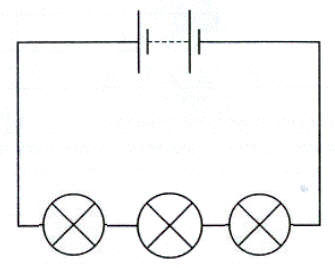Bulbs.com

243 Stafford St.

Worcester, MA 01603

Tel. 1 (888) 455-2800

Fax 1.508.363.2900

www.bulbs.comA beginner's guide to electrical circuits.
Learning CenterLighting Basics

# How Electrical Circuits Work

## Basic Circuits

An electric circuit is an unbroken path along which an electric current exists and/or is able to flow. A simple electrical circuit consists of a power source, two conducting wires (one end of each being attached to each terminal of the cell), and a small lamp to which the free ends of the wires leading from the cell are attached.

When the connections are made properly, the circuit will “close” and current will flow through the circuit and light the lamp.A simple electrical circuit

Once one of the wires is removed from the power source or a “break” is made in the flow, the circuit is now “open” and the lamp will no longer light.

In practical application, circuits are “opened” by such devices as switches, fuses, and circuit breakers. Two general circuit classifications are series and parallel.

The elements of a series circuit are connected end to end; the same current flows through its parts one after another.

## Series Circuits

In a series circuit , the current through each of the components is the same, and the voltage across the components is the sum of the voltages across each component.An example of a Series Circuit

## Parallel Circuits

In a parallel circuit, the voltage across each of the components is the same, and the total current is the sum of the currents through each component.

If two or more components are connected in parallel they have the same potential difference ( voltage) across their ends. The potential differences across the components are the same in magnitude, and they also have identical polarities. The same voltage is applicable to all circuit components connected in parallel.

If each bulb is wired to the battery in a separate loop, the bulbs are said to be in parallel.An example of a Parallel Circuit.

## Circuit Example

Consider a very simple circuit consisting of four light bulbs and one 6 V battery. If a wire joins the battery to one bulb, to a second bulb, to a third bulb, then back to the battery, in one continuous loop, the bulbs are said to be in series. If the three light bulbs are connected in series, the same current flows through all of them, and the voltage drop is 1.5 V across each bulb and that may not be sufficient to make them glow.If the light bulbs are connected in parallel, the current flowing through the light bulbs combine to form the current flowing in the battery, while the voltage drop is 6.0 V across each bulb and they all glow.

In a series circuit, every device must function for the circuit to be complete. One bulb burning out in a series circuit breaks the circuit. In parallel circuits, each light has its own circuit, so all but one light could be burned out, and the last one will still function.